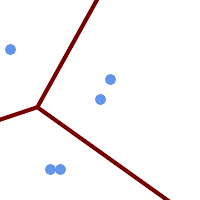## Name

ST_VoronoiLines — Returns the boundaries between the cells of the Voronoi diagram constructed from the vertices of a geometry.

## Synopsis

`geometry ST_VoronoiLines(` g1 geometry , tolerance float8 , extend_to geometry `)`;

## Description

ST_VoronoiLines computes a two-dimensional Voronoi diagram from the vertices of the supplied geometry and returns the boundaries between cells in that diagram as a MultiLineString. Returns null if input geometry is null. Returns an empty geometry collection if the input geometry contains only one vertex. Returns an empty geometry collection if the extend_to envelope has zero area.

Optional parameters:

• 'tolerance' : The distance within which vertices will be considered equivalent. Robustness of the algorithm can be improved by supplying a nonzero tolerance distance. (default = 0.0)

• 'extend_to' : If a geometry is supplied as the "extend_to" parameter, the diagram will be extended to cover the envelope of the "extend_to" geometry, unless that envelope is smaller than the default envelope (default = NULL, default envelope is boundingbox of input geometry extended by about 50% in each direction).

Availability: 2.3.0 - requires GEOS >= 3.5.0.

## ExamplesVoronoi lines with tolerance of 30 units ```SELECT ST_VoronoiLines(geom, 30) As geom FROM (SELECT 'MULTIPOINT (50 30, 60 30, 100 100,10 150, 110 120)'::geometry As geom ) As g``` ``` -- ST_AsText output MULTILINESTRING((135.555555555556 270,36.8181818181818 92.2727272727273),(36.8181818181818 92.2727272727273,-110 43.3333333333333),(230 -45.7142857142858,36.8181818181818 92.2727272727273)) ```